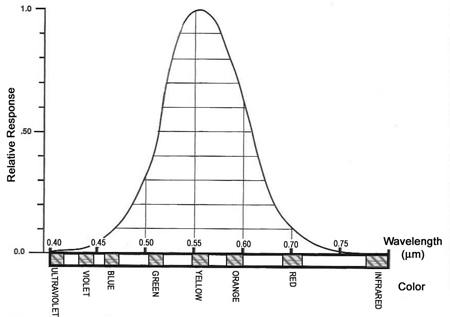# Explanation of light from Optical Engineering Fundamentals, Second Edition

Excerpt from Optical Engineering Fundamentals, Second Edition

## Light: an Elusive Topic

In order to begin to understand the field of optical engineering and the function of the optical engineer, it is essential that we first develop some sense of what light is and how it behaves. It has been the experience of many (including myself) that this understanding need be neither comprehensive nor precisely correct to be valuable to the optical engineer in the execution of his day-to-day responsibilities. After all, as we have seen in the previous chapter, many of the great minds throughout history have made significant contributions to the science of optics while maintaining incomplete, or in some cases completely erroneous, theories regarding the nature and behavior of light.

## Understanding Light

First, we can safely and correctly state that light is energy. We know that light is electromagnetic energy and that, in terms of wavelength, it represents a very small part of the broad electromagnetic spectrum (see Fig. 3.1). It will be helpful if we pursue our understanding of the term wavelength a bit further at this point. In one general and very useful definition, light is that portion of the electromagnetic spectrum that the human visual system (the eye) is capable of detecting. That is to say, light is the electromagnetic energy that we can see. Under normal daytime conditions, the eye has a maximum sensitivity to light with a wavelength of 0.56 micrometers (µm). Let us convert this number (0.56 µm) to a more meaningful and comprehensible form. A meter represents a length of about 40 inches, or a little more than one yard. A millimeter is 1/1000th of a meter, or about the thickness of 10 pages in this book. A micrometer (often referred to as a micron) is 1/1000 of a millimeter, and the peak wavelength for visible light is about one half of that. Therefore, one wavelength of visible light has a length approximately equal to 1/200 the thickness of a single page in this book.Figure 3.1 Wavelengths of the electromagnetic, optical, and visible spectra. (Click to enlarge.)

As we continue the study of optics we will find that the dimensions we encounter are most often represented by either very large or, as is the case for wavelength, very small numbers. In order to deal with these numbers it is helpful to understand and to use the system of scientific notation which incorporates the use of exponents. In its simplest form, this method presents us with a number between 1 and 10, followed by a notation indicating how many places the decimal point must be moved either to the left (-) or to the right (+). In this case, we might say that the peak visible wavelength is equal to 5.6 × 10-4 (0.00056) mm. For those readers who are not yet thinking and working in metric terms, this would convert to 2.2 × 10-5 (0.000022) in. As the first of many words of advice to the reader and would-be optical engineer, do make the conversion to thinking and working in the metric system. It will be most helpful and will enhance your effectiveness in nearly all areas of optical engineering.

As the wavelength of the energy (light) being collected by the eye increases or decreases, the color of that light as perceived by the eye will change. At the peak wavelength of 0.56 µm, the light will be seen as yellow. When the wavelength decreases to about 0.50 µm, the light appears to be green, while at 0.48 µm the color we see is blue. Moving in the other direction from the peak wavelength, at a wavelength of 0.60 µm, the light appears to be orange, and then, as the wavelength reaches 0.65 µm, we will see the light as red. This is what we refer to as the visible spectrum, ranging from violet (0.40 µm) to red (0.75 µm), with a peak sensitivity to the color yellow, at a wavelength of 0.56 µm. The visible spectrum, and its relationship to the electromagnetic spectrum, are shown in Fig. 3.1. Figure 3.2 shows the relative sensitivity of the eye as a function of wavelength, i.e., color.Figure 3.2 Spectral sensitivity of the human visual system. (Click to enlarge.)
Citation:

B. Walker, Optical Engineering Fundamentals, Second Edition, SPIE Press, Bellingham, WA (2008).